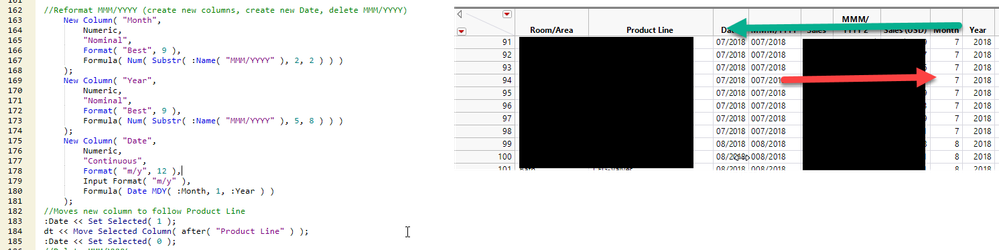The World Statistics Day celebration continues here in the Community. We all need reliable data for sound decision making. Do you have a data source that you trust most? Head over to Discussions to tell us about it.
Choose Language Hide Translation Bar
Highlighted

## Date: Date Type, Modeling Type and Format -> How to fix MMM/YYYY

Data in format MMM/YYYY (001/2019, 002/2019, ...012/2019) is being imported as Date Type Character, Modeling Type Nominal.
I'm trying to get JMP to recognize this as a date.  The final format isn't import per se.  I show a format below just as an example.

I've tried scripting two ways to fix with no luck. The column name is "MMM/YYYY"

[fail #1]

``````names default to here(1);
dt = current data table();
dt<< column(:"MMM/YYYY",Numeric,Continous,Format( "m/d/y", 10));``````

[fail #2]

``````names default to here(1);
dt = current data table();
:"MMM/YYYY"() << Date Type(Numeric) << Modeling Type(Continous) <<Format( "m/d/y", 10);``````

1 ACCEPTED SOLUTION

Accepted Solutions
Highlighted

## Re: Date: Date Type, Modeling Type and Format -> How to fix MMM/YYYY

The script below demonstrates that a simple Informat() will work, or you can strip off the leading zero and use Informat() .

``````New Column( "Date",
Numeric,
"Continuous",
Format( "m/y", 12 ),
Formula( Informat( Right( :Name("MMM/YYYY"), 8 ), "m/y" ) )
);

New Column( "Date2",
Numeric,
"Continuous",
Format( "m/y", 12 ),
Formula( Informat(  :Name("MMM/YYYY") , "m/y" ) )
);

``````
2 REPLIES 2
Highlighted

## Re: Date: Date Type, Modeling Type and Format -> How to fix MMM/YYYY

This code works, but it seems like there should be a simplier way.

``````//Reformat MMM/YYYY (create new columns, create new Date, delete MMM/YYYY)
New Column( "Month",
Numeric,
"Nominal",
Format( "Best", 9 ),
Formula( Num( Substr( :Name( "MMM/YYYY" ), 2, 2 ) ) )
);
New Column( "Year",
Numeric,
"Nominal",
Format( "Best", 9 ),
Formula( Num( Substr( :Name( "MMM/YYYY" ), 5, 8 ) ) )
);
New Column( "Date",
Numeric,
"Continuous",
Format( "m/y", 12 ),
Input Format( "m/y" ),
Formula( Date MDY( :Month, 1, :Year ) )
);
//Moves new column to follow Product Line
:Date << Set Selected( 1 );
dt << Move Selected Column( after( "Product Line" ) );
:Date << Set Selected( 0 );``````Highlighted

## Re: Date: Date Type, Modeling Type and Format -> How to fix MMM/YYYY

The script below demonstrates that a simple Informat() will work, or you can strip off the leading zero and use Informat() .

``````New Column( "Date",
Numeric,
"Continuous",
Format( "m/y", 12 ),
Formula( Informat( Right( :Name("MMM/YYYY"), 8 ), "m/y" ) )
);

New Column( "Date2",
Numeric,
"Continuous",
Format( "m/y", 12 ),
Formula( Informat(  :Name("MMM/YYYY") , "m/y" ) )
);

``````
Article Labels

There are no labels assigned to this post.